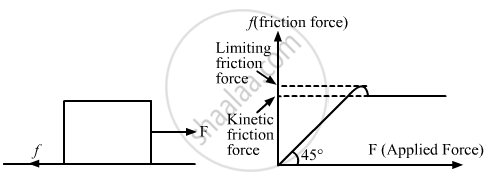Department of Pre-University Education, KarnatakaPUC Karnataka Science Class 11
Advertisement Remove all ads

# A Block is Placed on a Rough Floor and a Horizontal Force F Is Applied on It. the Force of Friction F By the Floor on the Block is Measured for Different Values Of F And a Graph - Physics

Answer in Brief

A block is placed on a rough floor and a horizontal force F is applied on it. The force of friction f by the floor on the block is measured for different values of F and a graph is plotted between them.
(a) The graph is a straight line of slope 45°.
(b) The graph is a straight line parallel to the F-axis.
(c) The graph is a straight line of slope 45° for small F and a straight line parallel to the F-axis for large F.
(d) There is a small kink on the graph.

Advertisement Remove all ads

#### Solution

(c) The graph is a straight line of slope 45° for small F and a straight line parallel to the F-axis for large F.
(d) There is a small kink on the graph.When force F is applied on the block, the force of friction f comes into play. As we increase the  applied F,  the static friction force adjusts itself to become  (equal) to the applied force F  and goes upto  its maximum value equal to limiting friction force.After this ,it is treated as a constant force (i.e . now its value does not change until and unless the body starts moving). If the applied force F is greater than the limiting friction force, then the kinetic friction force comes into play at that time. The kinetic friction force is always less than the limiting friction force.

Is there an error in this question or solution?
Advertisement Remove all ads

#### APPEARS IN

HC Verma Class 11, 12 Concepts of Physics 1
Chapter 6 Friction
MCQ | Q 4 | Page 97
Advertisement Remove all ads
Advertisement Remove all ads
Share
Notifications

View all notifications

Forgot password?